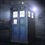# Trigo + Complex

Let $$A_1,A_2,...A_n$$ be a regular polygon of $$n$$ sides whose centre is origin $$O$$.Llet the complex numbers representing the vertices $$A_1,A_2.....A_n$$be$$z_1,z_2....z_n$$ respectively.The radius is of length unity.Then, $\prod_{n=2}^{n}|A_1A_n|=\mathbb{?}$5 years, 8 months ago

This discussion board is a place to discuss our Daily Challenges and the math and science related to those challenges. Explanations are more than just a solution — they should explain the steps and thinking strategies that you used to obtain the solution. Comments should further the discussion of math and science.

When posting on Brilliant:

• Use the emojis to react to an explanation, whether you're congratulating a job well done , or just really confused .
• Ask specific questions about the challenge or the steps in somebody's explanation. Well-posed questions can add a lot to the discussion, but posting "I don't understand!" doesn't help anyone.
• Try to contribute something new to the discussion, whether it is an extension, generalization or other idea related to the challenge.

MarkdownAppears as
*italics* or _italics_ italics
**bold** or __bold__ bold
- bulleted- list
• bulleted
• list
1. numbered2. list
1. numbered
2. list
Note: you must add a full line of space before and after lists for them to show up correctly
paragraph 1paragraph 2

paragraph 1

paragraph 2

[example link](https://brilliant.org)example link
> This is a quote
This is a quote
    # I indented these lines
# 4 spaces, and now they show
# up as a code block.

print "hello world"
# I indented these lines
# 4 spaces, and now they show
# up as a code block.

print "hello world"
MathAppears as
Remember to wrap math in $$ ... $$ or $ ... $ to ensure proper formatting.
2 \times 3 $2 \times 3$
2^{34} $2^{34}$
a_{i-1} $a_{i-1}$
\frac{2}{3} $\frac{2}{3}$
\sqrt{2} $\sqrt{2}$
\sum_{i=1}^3 $\sum_{i=1}^3$
\sin \theta $\sin \theta$
\boxed{123} $\boxed{123}$

Sort by:

Let the circumradius be $R$. Now we consider all $z$ that satisfy the circle and write a certain expansion:

$z^n-R^n=(z-R)(z^{n-1} + Rz^{n-2}+Rz^{n-3} + \dots + zR^{n-2}+R^{n-1})$

Then we have $z_1=R$ and $,z_2,z_3\dots z_n$ as roots of $(z^{n-1} + Rz^{n-2}+Rz^{n-3} + \dots + zR^{n-2}+R^{n-1})$ , So we can write :

$(z-z_2)(z-z_3) \dots (z-z_n)=z^{n-1} + Rz^{n-2}+Rz^{n-3} + \dots + zR^{n-2}+R^{n-1})$

Since $z_1=R$ is one of the $z$'s , we substitute $z=R$ in RHS . Thus the equation becomes:

$|(z-z_1)||(z-z_2)||(z-z_3)| \dots |(z-z_n)|=nR^{n-1} \dots (1)$

To calculate $R$ we use cosine rule and get $R=\dfrac{1}{2\sin\left(\dfrac{\pi}{n}\right)}$. So finally

$\displaystyle\prod_{i=2}^n |A_1A_n|=\dfrac{n}{2\sin^{n-1}\left(\dfrac{\pi}{n}\right)}$

Edit: The problem is edited and the circumradius is unity and not the side. Hence substituting $R=1$ in $(1)$ we get:

$\displaystyle\prod_{i=2}^n |A_1A_n|=n\times 1^{n-1} = \boxed{n}$

- 5 years, 8 months ago

Answer is $n$.

- 5 years, 8 months ago

Are you sure that the side equals unity? Or the circumradius?

- 5 years, 8 months ago

Oh!Sorry!R=1!

- 5 years, 8 months ago

Yes , now the answer is $n$.

- 5 years, 8 months ago

I asked this question a while ago and Alan Yan wrote a nice solution

- 5 years, 8 months ago

- 5 years, 8 months ago

Thanks a lot sir!

- 5 years, 8 months ago